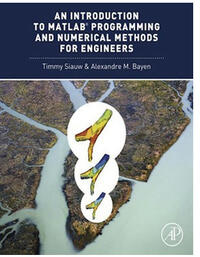# An Introduction to Matlab

The E7 class follows a curriculum outlined by the book below. Please refer to the book for detailed content of the class.

####An Introduction to MATLAB® Programming and Numerical Methods for Engineers

Timmy Siauw, Alexandre Bayen

Academic Press, 1st Edition, 2014, 340 p

ISBN: 9780124202283

Elsevier Website

Assuming no prior background in linear algebra or real analysis, An Introduction to MATLAB® Programming and Numerical Methods for Engineers enables you to develop good computational problem solving techniques through the use of numerical methods and the MATLAB® programming environment. Part One introduces fundamental programming concepts, using simple examples to put new concepts quickly into practice. Part Two covers the fundamentals of algorithms and numerical analysis at a level allowing you to quickly apply results in practical settings.

Contents:

• MATLAB Basics
• Variables and Basic Data Structures
• Functions
• Branching Statement
• Iteration
• Recursion
• Complexity
• Representation of Numbers
• Errors, Good Practices, and Debugging
• Visualization and Plotting
• Linear Algebra and Systems of Linear Equations
• Least Squares Regression
• Interpolation
• Series
• Root Finding
• Finite Differences and Numerical Differentiation
• Numerical Integration
• Ordinary Differential Equations